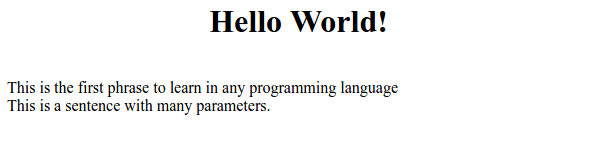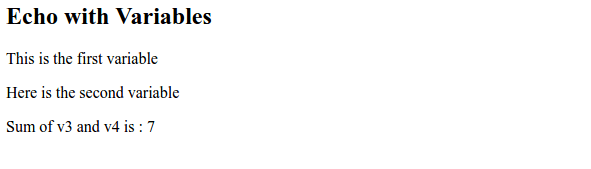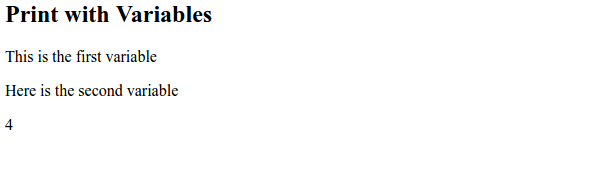PHP provides two methods of outputting data onto the screen. Those are PHP echo and print statements.

Both statements give an output in a similar manner. However, we shall explore their differences.

The PHP echo is a keyword in the language that returns no value after completion of execution. Print, on the other hand, returns 1 as its value after execution.

Echo is used frequently with many parameters. Print can only allow a single argument one at a time.  Also, the former is faster compared to the latter.

## PHP Echo :

It may be used with parenthesis, depending on the preference of the developer.

``````<?php

//Php echo
echo "<center><h1>Hello World!</h1></center>";
echo "<br>";
echo "This is the first phrase to learn in any programming language";
echo "<br>";
echo "This ", "is ", "a ", "sentence ", "with ", "many ", "parameters.";
?>``````

##PHP echo with variables:

We can use the php variables in echo statements. We can also do the dynamic calculations in php echo. Here is the example.

``````<?php

//PHP echo statements with variables
\$v1 = "This is the first variable";
\$v2 = "Here is the second variable";
\$v3 = "3";
\$v4 = "4";

echo "<h2>Echo with Variables</h2>";
echo "<p>\$v1</p>";
echo "<p>\$v2</p>";
echo "Sum of v3 and v4 is : ",\$v3 + \$v4;
?>``````

Output :

##PHP print statement :

PHP print statement is used to outputting the data on the screen.

The print statement, may also be used with the parentheses: print () or print.

This is how it is used :

``````<?php
// PHP print example
print "<h2>Lets learn PHP</h2>";
print "I am enthusiastic about programming <br>";
print("I will be a great at programming");
?>``````

Output :Like PHP echo, we can also use the dynamic variables in PHP print. With variables, this is how the print statement is used :

``````<?php

//PHP print statements with variables
\$v1 = "This is the first variable";
\$v2 = "Here is the second variable";
\$v3 = "3";
\$v4 = "4";

print "<h2>Print with Variables</h2>";
print "<p>\$v1</p>";
print "<p>\$v2</p>";
print (\$v3 + \$v4);
?>``````

Output :Happy Learning 🙂